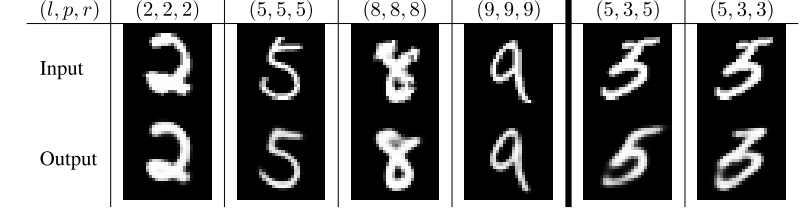# 矢量的胶囊输入和输出是如何被计算的

$$v_j = \frac{||s_j||^2}{1+||s_j||^{2}} \frac{s_j}{||s_j||}$$

$v_{j}$ 就是第 $j$ 个胶囊的矢量输出，$s_{j}$ 是总体的输入

$$s_{j} = \sum_{i} c_{ij} \hat{u}_{j|i}$$

$$\hat{u}_{j|i} = W_{ij}u_{i}$$

$c_{ij}$是动态路由过程之中的参数。

$$c_{ij} = \frac{exp(b_{ij})}{\sum_{k}exp(b_{ik})}$$

# 边缘分布

Capsule使用一个矢量来表达胶囊实体存在的可能性。对于最顶层的胶囊来说，当且仅当这一位在图片中出现的时候，对应的第k类将会有一个长的实例化向量。为了其能够适用于不同的类，对于每一个类所对应的胶囊，我们将会使用一个单独的边缘分布$L_{k}$：

$$L_{k} = T_{k} \max(0,m^{+}-||v_{k}||)^{2} + \lambda (1-T_{k}) \max(0,||v_{k}||-m^{-})^2$$

# CapsNet架构## 基于正则化的空间信息重建Baseline - - 0.39 8.1
CapsNet 1 no 0.34+-0.0032 -
CapsNet 1 yes 0.29+-0.011 7.5
CapsNet 3 no 0.35+-0.036 -
CapsNet 3 yes 0.25+-0.005 5.2